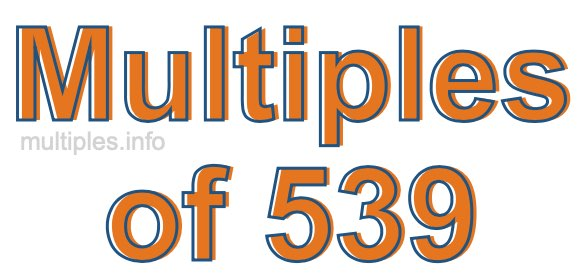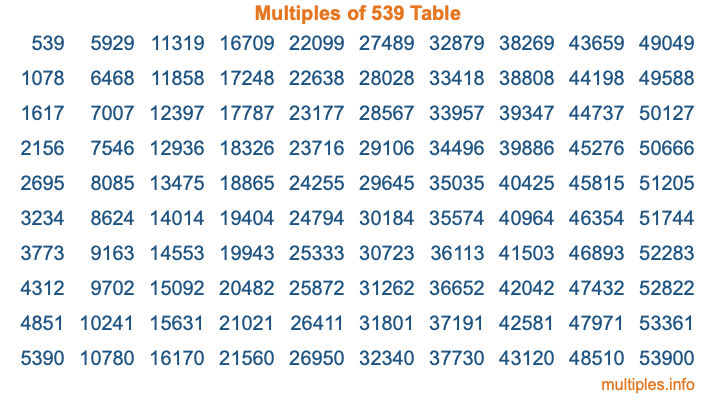Multiples of 539Welcome to the Multiples of 539 page. Here we will first teach you everything you will ever need to know about the multiples of 539, and then give you a study guide summary of everything we taught you to make sure you remember it all. Use this page to look up facts and learn information about the multiples of 539. This page will make you a multiples of five hundred thirty-nine expert!

Definition of Multiples of 539
Multiples of 539 are all the numbers that when divided by 539 equal an integer. Each of the multiples of 539 are called a multiple. A multiple of 539 is created by multiplying 539 by an integer.

Therefore, to create a list of multiples of 539, you start with 1 multiplied by 539, then 2 multiplied by 539, then 3 multiplied by 539, and so on for as long as you want. Thus, the list of the first five multiples of 539 is 539, 1078, 1617, 2156, and 2695. To see a larger list of multiples of 539, see the printable image of Multiples of 539 further down on this page. We also have a category where you can choose any nth multiple of 539.

Multiples of 539 Checker
The Multiples of 539 Checker below checks to see if any number of your choice is a multiple of 539. In other words, it checks to see if there is any number (integer) that when multiplied by 539 will equal your number. To do that, we divide your number by 539. If the the quotient is an integer, then your number is a multiple of 539.

Is  a multiple of 539?

Least Common Multiple of 539 and ...
A Least Common Multiple (LCM) is the lowest multiple that two or more numbers have in common. This is also called the smallest common multiple or lowest common multiple and is useful to know when you are adding our subtracting fractions. Enter one or more numbers below (539 is already entered) to find the LCM.

Check out our LCM Calculator if you need more details about the Least Common Multiple or if you need the LCM for different numbers for adding and subtraction fractions.

nth Multiple of 539
As we stated above, 539 is the first multiple of 539, 1078 is the second multiple of 539, 1617 is the third multiple of 539, and so on. Enter a number below to find the nth multiple of 539.

th multiple of 539

Multiples of 539 vs Factors of 539
539 is a multiple of 539 and a factor of 539, but that is where the similarities end. All postive multiples of 539 are 539 or greater than 539. All positive factors of 539 are 539 or less than 539.

Below is the beginning list of multiples of 539 and the factors of 539 so you can compare:

Multiples of 539: 539, 1078, 1617, 2156, 2695, etc.

Factors of 539: 1, 7, 11, 49, 77, 539

As you can see, the multiples of 539 are all the numbers that you can divide by 539 to get a whole number. The factors of 539, on the other hand, are all the whole numbers that you can multiply by another whole number to get 539.

It's also interesting to note that if a number (x) is a factor of 539, then 539 will also be a multiple of that number (x).

Multiples of 539 vs Divisors of 539
The divisors of 539 are all the integers that 539 can be divided by evenly. Below is a list of the divisors of 539.

Divisors of 539: 1, 7, 11, 49, 77, 539

The interesting thing to note here is that if you take any multiple of 539 and divide it by a divisor of 539, you will see that the quotient is an integer.

Multiples of 539 Table
Below is an image of the first 100 multiples of 539 in a table. The table is in chronological order, column by column. The first column has the first ten multiples of 539, the second column has the next ten multiples of 539, and so on.The Multiples of 539 Table is also referred to as the 539 Times Table or Times Table of 539. You are welcome to print out our table for your studies.

Negative Multiples of 539
Although not often discussed or needed in math, it is worth mentioning that you can make a list of negative multiples of 539 by multiplying 539 by -1, then by -2, then by -3, and so on, to get the following list of negative multiples of 539:

-539, -1078, -1617, -2156, -2695, etc.

Multiples of 539 Summary
Below is a summary of important Multiples of 539 facts that we have discussed on this page. To retain the knowledge on this page, we recommend that you read through the summary and explain to yourself or a study partner why they hold true.

There are an infinite number of multiples of 539.

A multiple of 539 divided by 539 will equal a whole number.

539 divided by a factor of 539 equals a divisor of 539.

The nth multiple of 539 is n times 539.

The largest factor of 539 is equal to the first positive multiple of 539.

539 is a multiple of every factor of 539.

539 is a multiple of 539.

A multiple of 539 divided by a divisor of 539 equals an integer.

539 divided by a divisor of 539 equals a factor of 539.

Any integer times 539 will equal a multiple of 539.

Multiples of a Number
Here you can get the multiples of another number, all with the same attention to detail as we did for multiples of 539 on this page.

Multiples of
Multiples of 540
Did you find our page about multiples of five hundred thirty-nine educational? Do you want more knowledge? Check out the multiples of the next number on our list!